# CSCI 3328 Object Oriented Programming in C Chapter

• Slides: 20CSCI 3328 Object Oriented Programming in C# Chapter 5: C# Control Statement – Part II Xiang Lian The University of Texas Rio Grande Valley Edinburg, TX 78539 xiang. [email protected] edu 1Objectives • In this chapter, you will: – Learn more control structures • Repetition statements – for, do … while • Selection – switch – Know how to use break and continue statements to terminate the loop or current iteration – Learn how to use logical operators in C# 2Repetition Structure • Visual C# provides 3 repetition statements – while – for – do … while 3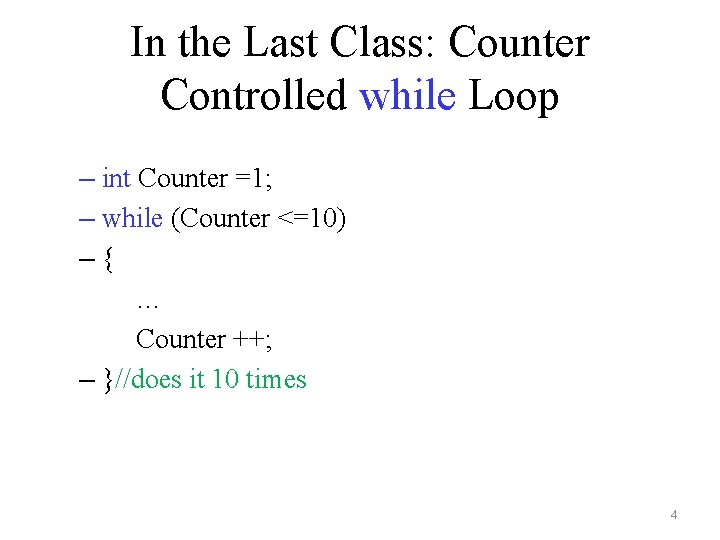In the Last Class: Counter Controlled while Loop – int Counter =1; – while (Counter <=10) –{ … Counter ++; – }//does it 10 times 4Example of for Repetition Statement • Output even numbers between 2 and 10 for (int counter = 2; counter<= 10; counter+=2) { Console. Write("{0} ", counter); Console. Write. Line(); } 5Discussions on for Repetition Statement • for (int counter = 2; counter <= 10; counter += 2) – Keywords: for – "int counter" declares a counter of integer data type • counter – control variable name • int – control variable type – Initial value of control variable – 2 – Final value of control variable – 10 6General Form of a for Statement • for (initialization; loop. Continuation. Condition; increment) { statement } 7Other Examples of for Statement (1) • Declaring the control variable before a for statement – int counter; for (counter = 2; counter <= 10; counter+=2) Console. Write("{0} ", counter); • Using expressions in the for statement –for (counter = x; counter <= 4*x*y; counter+=y/x) Console. Write("{0} ", counter); 8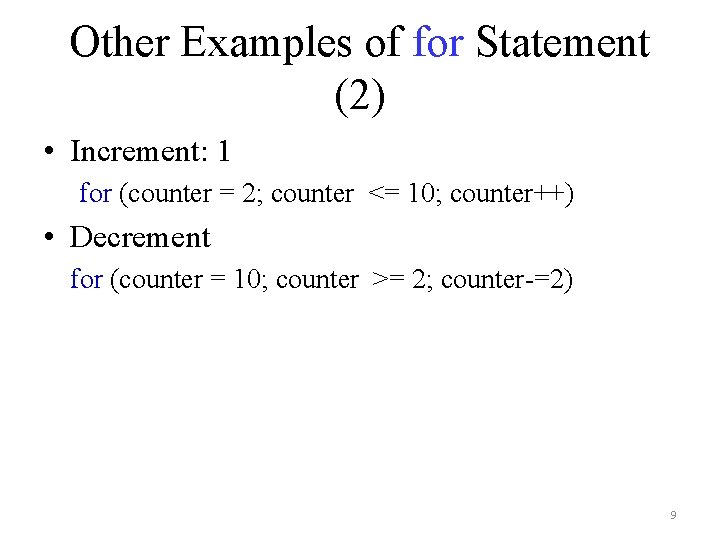Other Examples of for Statement (2) • Increment: 1 for (counter = 2; counter <= 10; counter++) • Decrement for (counter = 10; counter >= 2; counter-=2) 9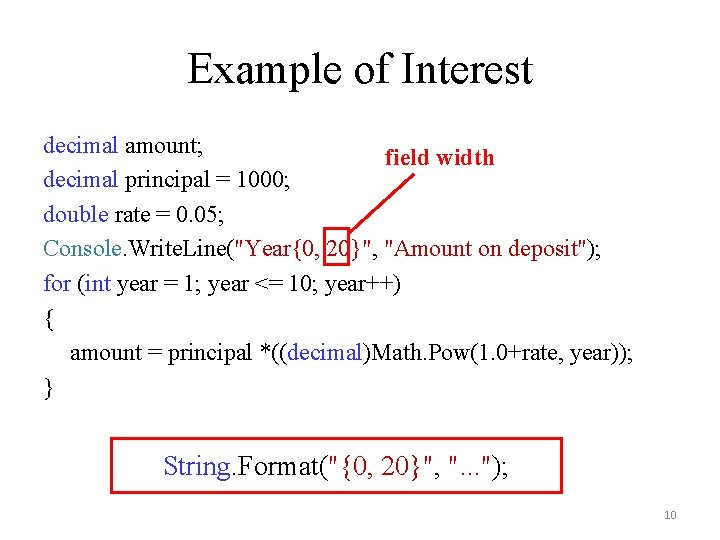Example of Interest decimal amount; field width decimal principal = 1000; double rate = 0. 05; Console. Write. Line("Year{0, 20}", "Amount on deposit"); for (int year = 1; year <= 10; year++) { amount = principal *((decimal)Math. Pow(1. 0+rate, year)); } String. Format("{0, 20}", ". . . "); 10do…while Repetition Statements • The loop body is always executed at least once int product = 1; do { product = product * 3; }while (product <=100); 11Use break to Terminate Repetition Statements for (int counter = 1; counter <=10; counter ++) { if (counter == 5) break; Console. Write("{0} ", counter); } // answer: 1 2 3 4 12Use continue to Terminate the Current Iteration for (int counter = 1; counter <=10; counter ++) { if (counter == 5) continue; Console. Write("{0} ", counter); } // answer: 1 2 3 4 6 7 8 9 10 13In the Last Class: Selection Structures in C# • if – single selection statement • if … else – double selection statement • switch – multiple selection statement [grade>=60] display "passed" [grade<60] 14Example of Multiple Selection Statement public void Calculate. Grade(int grade) { switch (grade/10) { case 9: case 10: Console. Write. Line("Grade: A"); break; case 8: Console. Write. Line("Grade: B"); break; case 7: Console. Write. Line("Grade: C"); break; case 6: Console. Write. Line("Grade: D"); break; default: Console. Write. Line("Grade: F"); break; } } // 90 -99 // 100 // 80 -89 // 70 -79 // 60 -69 // < 60 15Logical Operators • Conditional AND operator (&&) – if (gender == 'F' && age >=65) – senior. Females += 1; Expression 1 Expression 2 false true Expression 1 && Expression 2 false true 16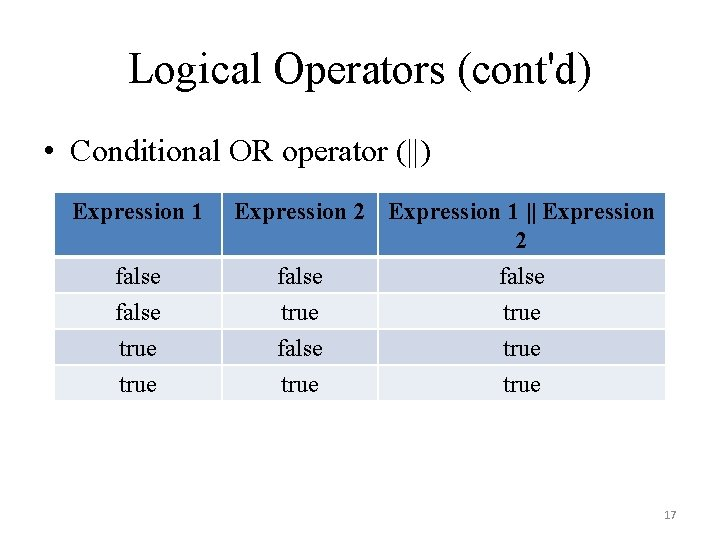Logical Operators (cont'd) • Conditional OR operator (||) Expression 1 false true Expression 2 Expression 1 || Expression 2 false true true 17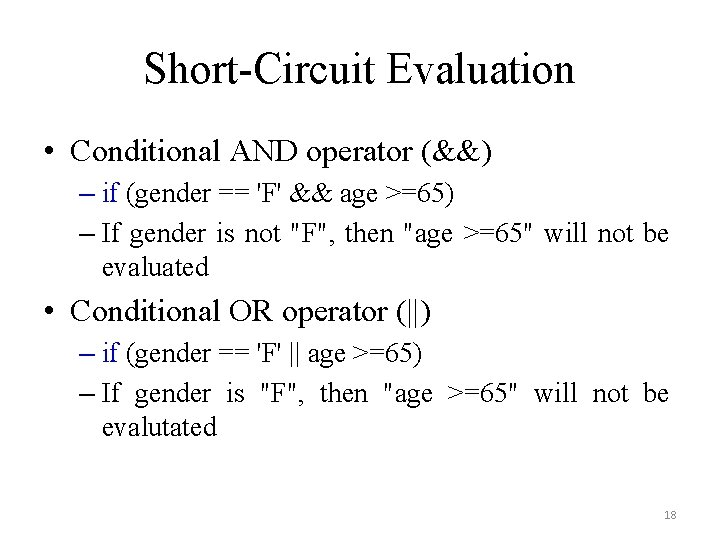Short-Circuit Evaluation • Conditional AND operator (&&) – if (gender == 'F' && age >=65) – If gender is not "F", then "age >=65" will not be evaluated • Conditional OR operator (||) – if (gender == 'F' || age >=65) – If gender is "F", then "age >=65" will not be evalutated 18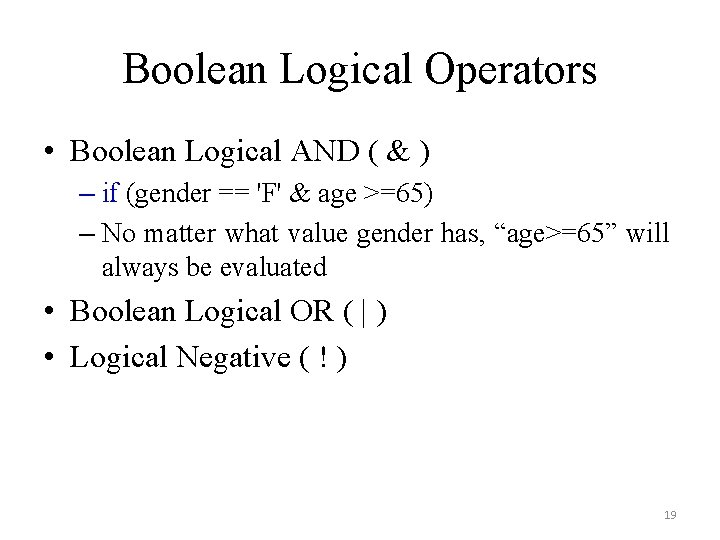Boolean Logical Operators • Boolean Logical AND ( & ) – if (gender == 'F' & age >=65) – No matter what value gender has, “age>=65” will always be evaluated • Boolean Logical OR ( | ) • Logical Negative ( ! ) 19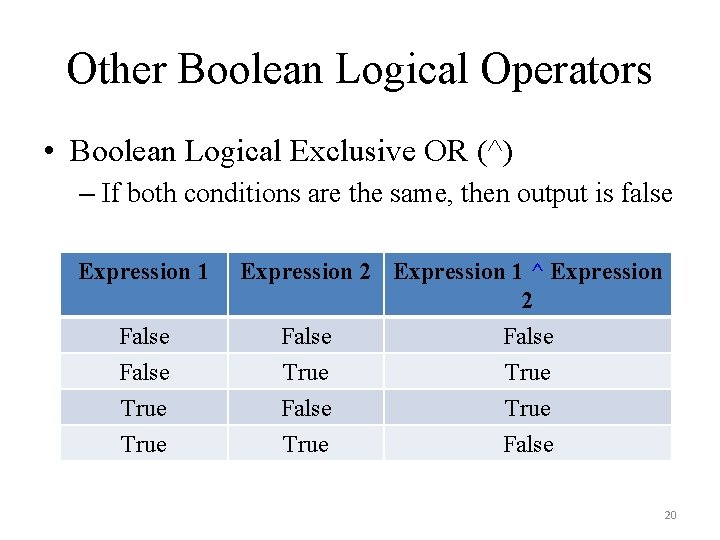Other Boolean Logical Operators • Boolean Logical Exclusive OR (^) – If both conditions are the same, then output is false Expression 1 False True Expression 2 Expression 1 ^ Expression 2 False True False 20